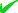News By Tag
Industry News
News By Location

Country(s)

Industry News

May 2023
TuMoSuSaFrThWe
30292827262524

# The Bernoulli Equation in Nanochannel Flow

Vanishing fluid viscosity induced by QED in nanochannels as the explanation of flow enhancement over Hagen-Poiseuille theory allows the frictionless Bernoulli equation to be a reasonably accurate measure of flow at the naonscale

The Bernoulli equation from QED induced charging of fluid atoms in nanochannels
YOUNGWOOD, Pa. - Jan. 30, 2014 - PRLog -- .
Introduction

Flow through nanochannels of CNTs is observed [1-5] to be 2-5 orders of magnitude higher than predicted by Hagen-Poiseuille equation. CNT stands for carbon nanotube. Finding origin in classical physics, the Hagen-Poiseuille equation assumes a no-slip condition of the fluid at the channel wall. However, to explain the disparity with experiment, the fluid is generally thought to slip at the wall. But slip is questionable because the calculated slip-lengths necessary to explain the high flow enhancement exceed the typical slip on non-wetting surfaces by 2 to 3 orders of magnitude. Hence, fluid slip at the channel wall is an unlikely explanation for the observed flow enhancement in nanochannels.
In this regard, flow enhancement is more likely caused by the size effect of QM that causes the viscosity of the fluid to vanish in nanochannels that otherwise does not occur at the macroscale. QM stands for quantum mechanics. Indeed, MD simulations for L-J potentials of liquid argon showed the viscosity to vanish because of the QM requirement that the atom does not have the heat capacity under the TIR confinement of the nanochannel to conserve frictional viscous heating by an increase in temperature. MD stands for molecular dynamics, L-J for Lennard-Jones, and TIR for total internal reflection. Instead, the viscous heat is conserved by the QED induced creation of EM radiation inside the nanochannel. QED stands for quantum electrodynamics and EM for electromagnetic. The EM radiation having sufficient Planck energy to ionize the fluid molecules induces a state of Coulomb repulsion among the fluid atoms as well as those on the channel wall. Atoms tend to separate from neighbors thereby causing the fluid viscosity to vanish. http://www.prlog.org/12271497-nanochannel-flow-by-quantum...

Bernoulli Equation
Vanishing viscosity suggests the flow in nanochannels should approach the frictionless flow given by the Bernoulli equation. The development of the Bernoulli equation from the QED energy equation in nanochannels is illustrated in the thumbnail. An incompressible fluid at the channel inlet in the Pi, Ti, Vi state leaves the channel in the outlet Po, To, Vo state. P stands for pressure T for temperature, and V for velocity. For a flow rate m, the power of the QED radiation created equal to the viscous heating is given by the mass flow rate m, and density ρ and heat capacity C if the fluid. Thus, QED radiation created by conserving the viscous heating inside the nanochannel ionizes the fluid molecules that otherwise is lost to the surroundings. Assuming an inlet plenum, the velocity Vi is negligible, while QM requires the heat capacity C to vanish under TIR confinement. Since viscous friction is a negligible fraction of the flow energy Pi / ρ , the QED radiation conserving viscous friction may be considered to vanish thereby giving the Bernoulli equation. For the channel flow area A, the mass flow rate m given by the Bernoulli equation approximates the flow in nanochannels.

Application to Nanochannels
In nanochannels, the application of the Bernoulli equation to flow measurements [1-5] from experiments on various fluids reported in the literature is compiled in . The compilation is especially relevant as the ratio Ω = M / B is given, where M is the measured flow and B the flow by the Bernoulli equation. Note the notation here differs from that in Table 1 of . For convenience, Reference 6 is available in “The Bernoulli Equation and Nanochannel Flow” at http://www.nanoqed.org , 2014.

Generally, Table 1 of  shows the ratio Ω < 1 as frictionless Bernoulli flow  upper bounds the flow in nanochannels. However, water at CNT radii of 3.5 nm [1, 2] and 0.8 nm  shows Ω > 1. For ethanol, isopropanol, decane, and hexane, the ratio Ω < 1. The data [1-3] for flow of water through CNTs is therefore questionable. Regardless, measured flow for Ω < 1 is reasonably close to 1 to support the argument that Bernoulli flow is a valid estimate of flow in nanochannels.

Conclusions
1. QED induced vanishing viscosity in nanochannels suggests the frictionless Bernoulli equation provides a reasonable estimate of flow at the nanoscale. The Bernoulli equation only requires the area A of the nanochannel, inlet pressure Pi, and the fluid ρ density, the simplicity of which avoids the complex and time consuming effort in performing MD simulations. Since standard MD programs do not provide valid solutions by QM, the effort is not only time consuming but meaningless. Even if valid MD solutions by QM are obtained, the Bernoulli equation is the far simpler method of computing the flow in nanochannels.

2. Experiments of flow measurements of water in nanochannels should be reviewed as the Bernoulli equation for frictionless flow cannot be exceeded.

References
 M. Majunder, et al., “Nanoscale hydrodynamics: Enhanced flow in carbon nanotubes,” Nature 438, 44, 2005.
 M. Majumder, et al., “Mass transport through carbon nanotube membranes in three different regimes: Ionic diffusion and gas and liquid flow,” ACS Nano, 5, 3867, 2011.
 J. Holt, et al., “Fast Mass Transport Through Sub–2-Nanometer Carbon Nanotubes,” Science, 312, 1034, 2006.
 F. Du, et al., “Membranes of vertically aligned superlong carbon nanotubes,” Langmuir, 27, 8437, 2011.
 T. Sisan and S. Lichter, “The end of nanochannels,” Microfluid-Nanofluid, 11, 787, 2011.
End
Source Email : QED Radiations : ***@gmail.com : Nanochannnel Flow, Bernoulli equaton, Quantum Mechanics, Qed, Viscous Heating : Science, Technology : Youngwood - Pennsylvania - United States : Reports
Account Email AddressAccount Phone NumberDisclaimer     Report Abuse
Page Updated Last on: Jan 31, 2014